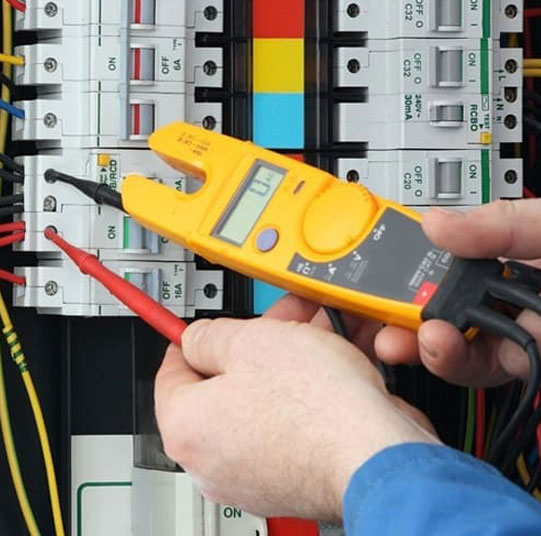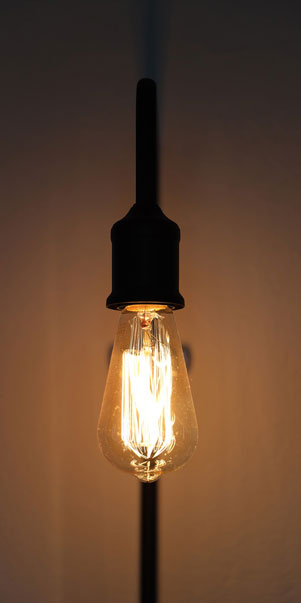# Power Factor Calculator

Power Factor
0.61

This is a high-end calculator used for calculating power factor.The general formula used in this calculation is PF=P/S, where P - watts, S=V×I - volt-amperes. Given below are the various formulas for calculating Power Factor.

## Power Triangle

You can calculate the power factor by using the power triangle. You can use the principles of trigonometry. Here is what you get -
P / S = cos φ
Because power factor is equal to the ratio between real and apparent power, Power factor = cos φ

#### Power Factor Formula

##### Single Phase

Power Factor, PF = P(W)(V(V) × I(A))
Apparent Power, S(VA) = V(V) × I(A)
Reactive Power, Q(VAR) = √(|S(VA)|2 – P(W)2)

##### Three Phase

Power Factor, PF = P(W) (√3 × V(V) × I(A))
Apparent Power, S(VA) = √3 × V(V) × I(A)
Reactive Power, Q(VAR) = √(|S(VA)|2 – P(W)2)## Power Factor Component Formulas

Real power is the power which is consumed in an AC circuit. This is also known as true power or active power. The measurement unit for real power is watts.

##### Real Power Formulas:
• P = V I (In DC circuits)
• P = VI Cosθ (in Single phase AC Circuits)
• P = √3 VL IL Cosθ or (in Three Phase AC Circuits)
• P = 3 VPh IPh Cosθ
• P = √ (S2 – Q2) or
• P =√ (VA2 – VAR2) or Real or True power = √ (Apparent Power2– Reactive Power2) or
• kW = √ (kVA2 – kVAR2)